# Midterm Test - Physical Science

Created
Best for live in-class or video conferencing lessonsStart teacher-led lesson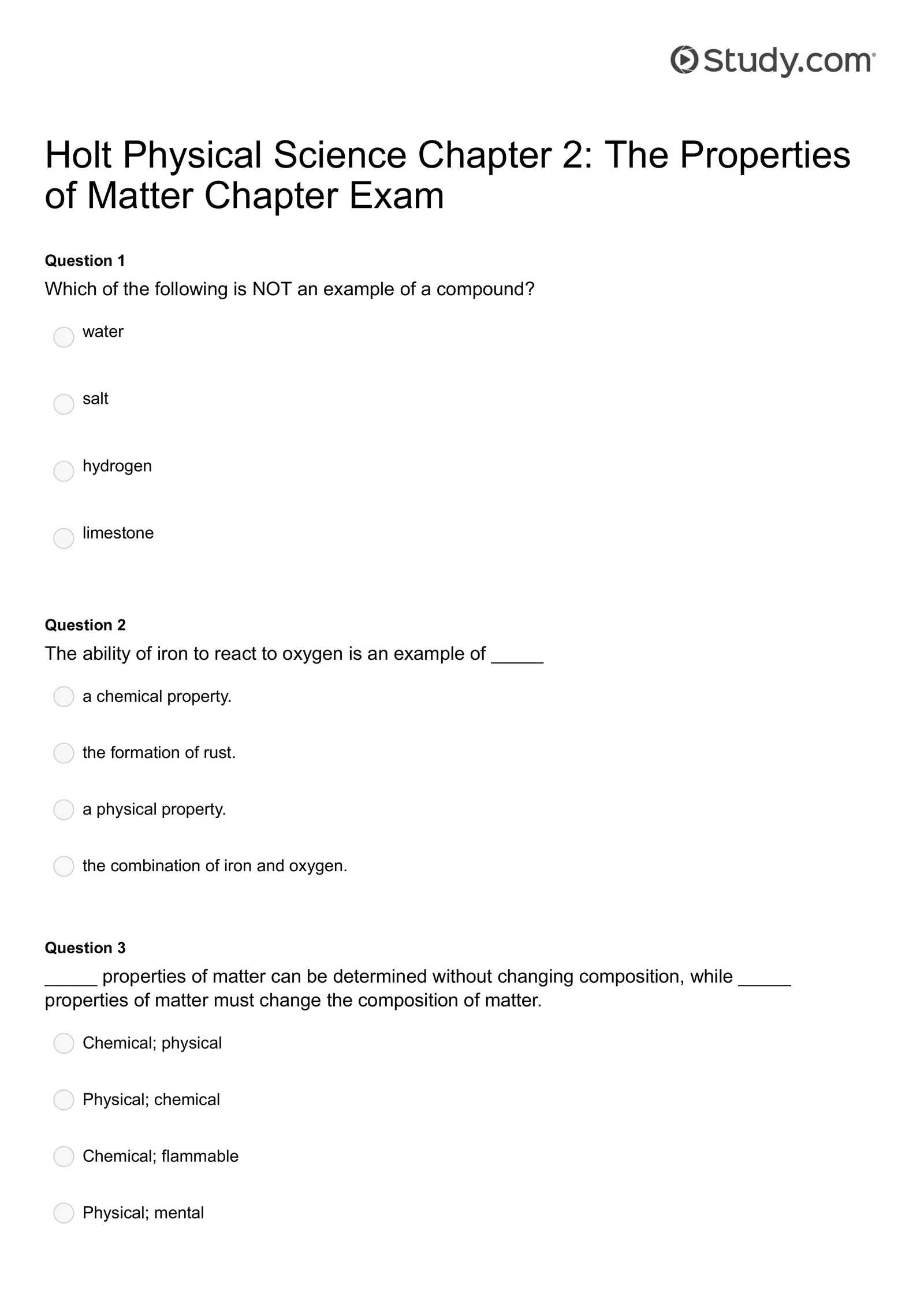Holt Physical Science Chapter 2: The Properties of Matter Chapter Exam Question 1 Which of the following is NOT an example of a compound? water salt hydrogen limestone Question 2 The ability of iron to react to oxygen is an example of _____ a chemical property. the formation of rust. a physical property. the combination of iron and oxygen. Question 3 _____ properties of matter can be determined without changing composition, while _____ properties of matter must change the composition of matter. Chemical; physical Physical; chemical Chemical; flammable Physical; mental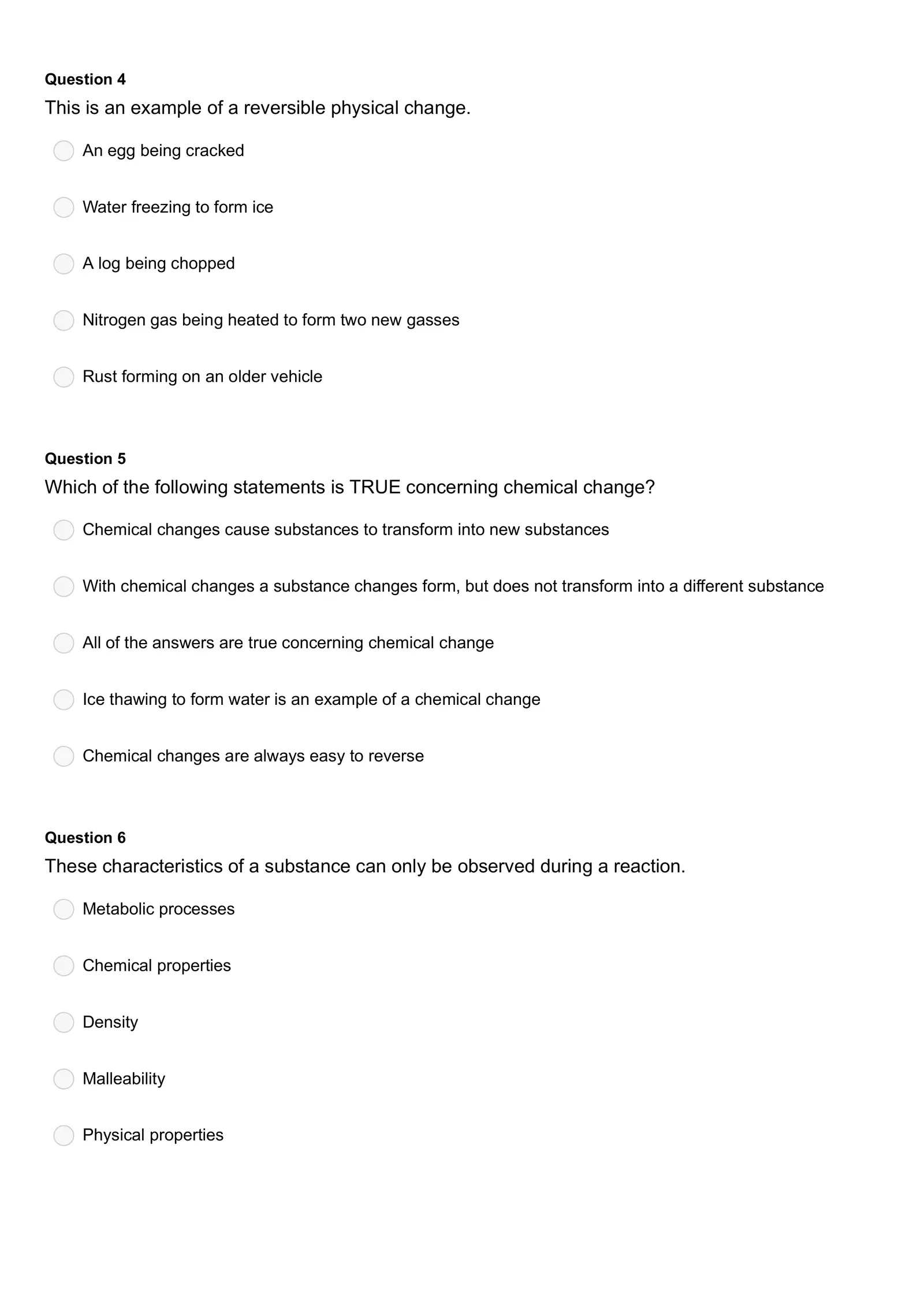Question 4 This is an example of a reversible physical change. An egg being cracked Water freezing to form ice A log being chopped Nitrogen gas being heated to form two new gasses Rust forming on an older vehicle Question 5 Which of the following statements is TRUE concerning chemical change? Chemical changes cause substances to transform into new substances With chemical changes a substance changes form, but does not transform into a different substance All of the answers are true concerning chemical change Ice thawing to form water is an example of a chemical change Chemical changes are always easy to reverse Question 6 These characteristics of a substance can only be observed during a reaction. Metabolic processes Chemical properties Density Malleability Physical properties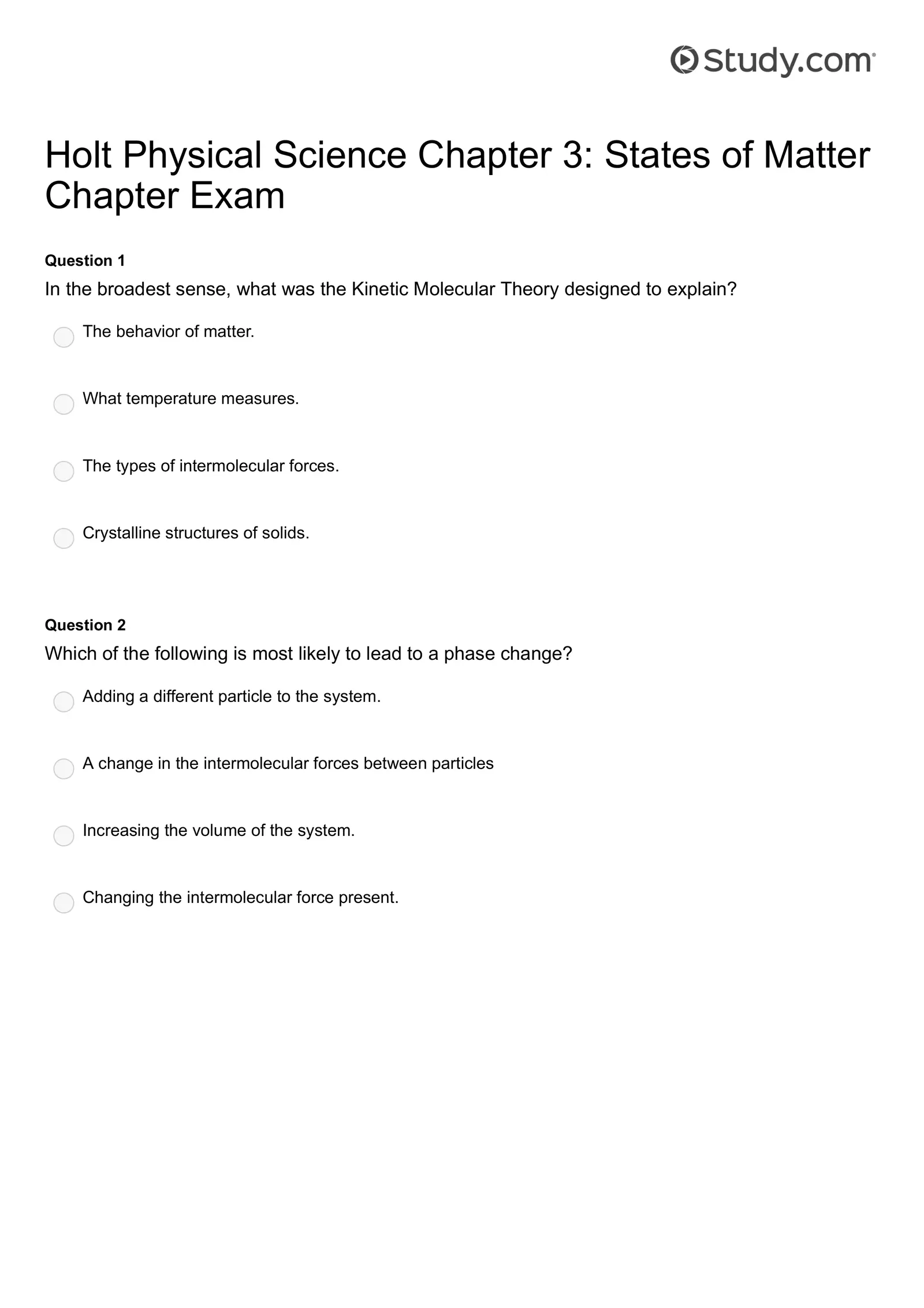Holt Physical Science Chapter 3: States of Matter Chapter Exam Question 1 In the broadest sense, what was the Kinetic Molecular Theory designed to explain? The behavior of matter. What temperature measures. The types of intermolecular forces. Crystalline structures of solids. Question 2 Which of the following is most likely to lead to a phase change? Adding a different particle to the system. A change in the intermolecular forces between particles Increasing the volume of the system. Changing the intermolecular force present.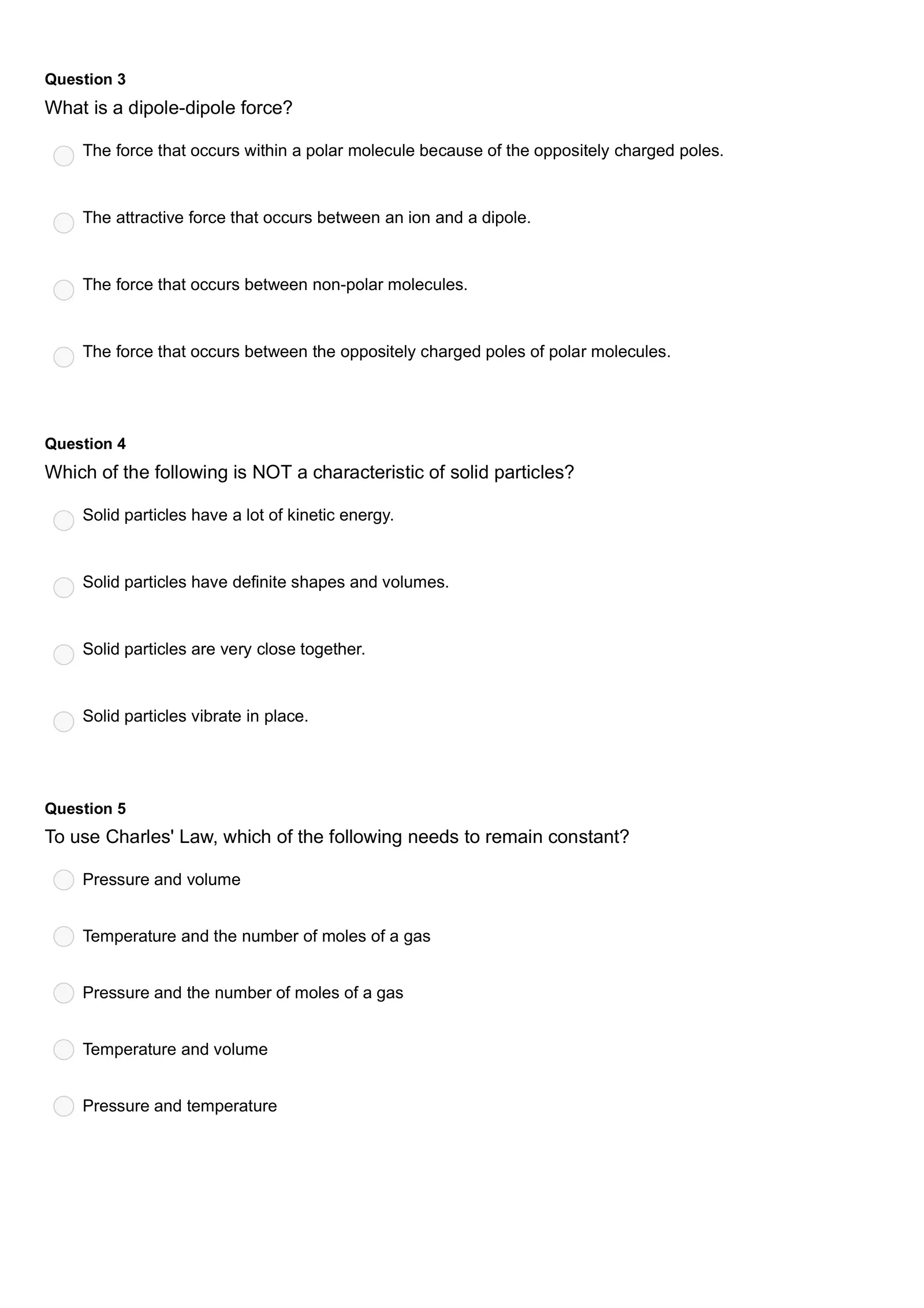Question 3 What is a dipole-dipole force? The force that occurs within a polar molecule because of the oppositely charged poles. The attractive force that occurs between an ion and a dipole. The force that occurs between non-polar molecules. The force that occurs between the oppositely charged poles of polar molecules. Question 4 Which of the following is NOT a characteristic of solid particles? Solid particles have a lot of kinetic energy. Solid particles have definite shapes and volumes. Solid particles are very close together. Solid particles vibrate in place. Question 5 To use Charles' Law, which of the following needs to remain constant? Pressure and volume Temperature and the number of moles of a gas Pressure and the number of moles of a gas Temperature and volume Pressure and temperature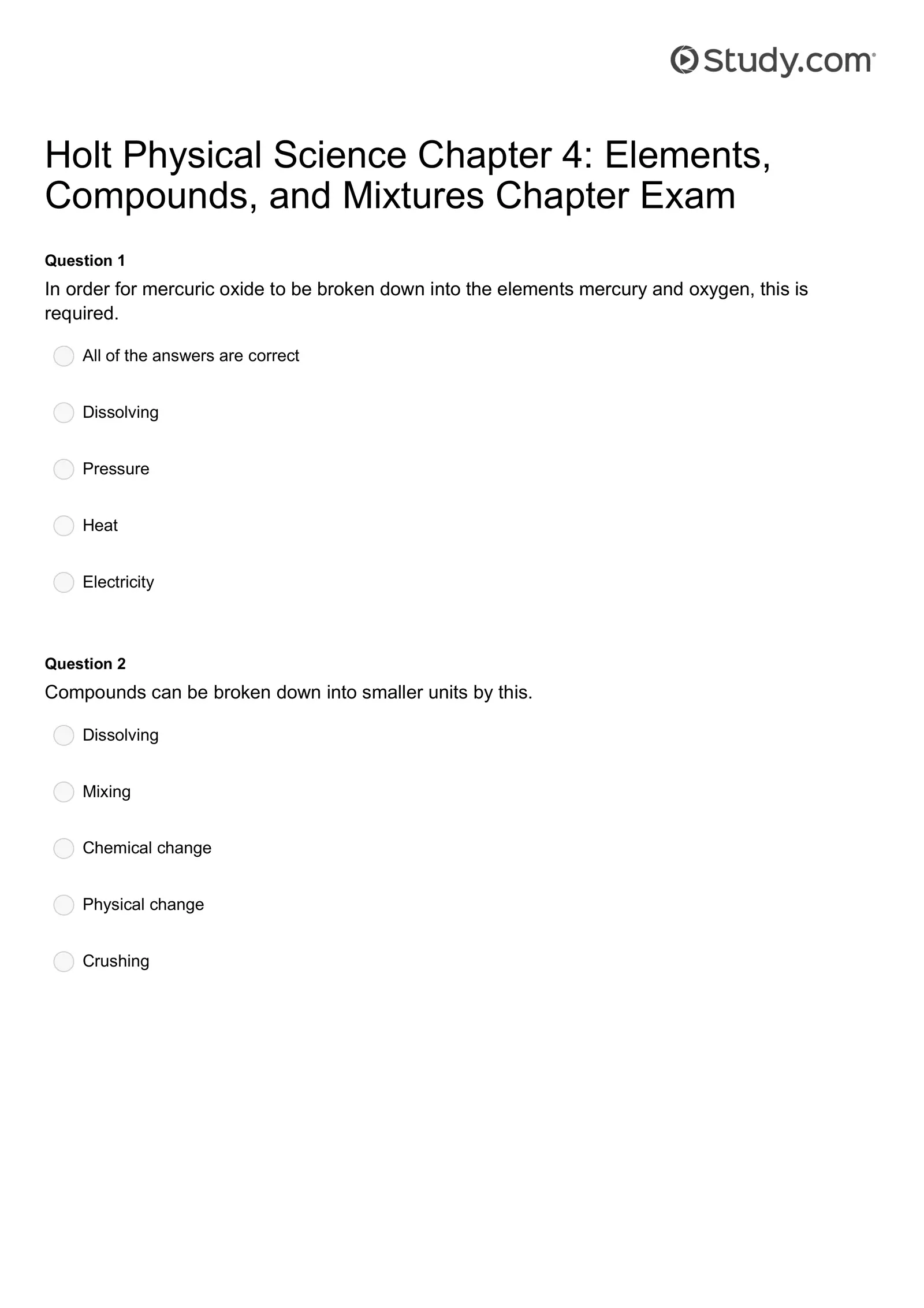Holt Physical Science Chapter 4: Elements, Compounds, and Mixtures Chapter Exam Question 1 In order for mercuric oxide to be broken down into the elements mercury and oxygen, this is required. All of the answers are correct Dissolving Pressure Heat Electricity Question 2 Compounds can be broken down into smaller units by this. Dissolving Mixing Chemical change Physical change Crushing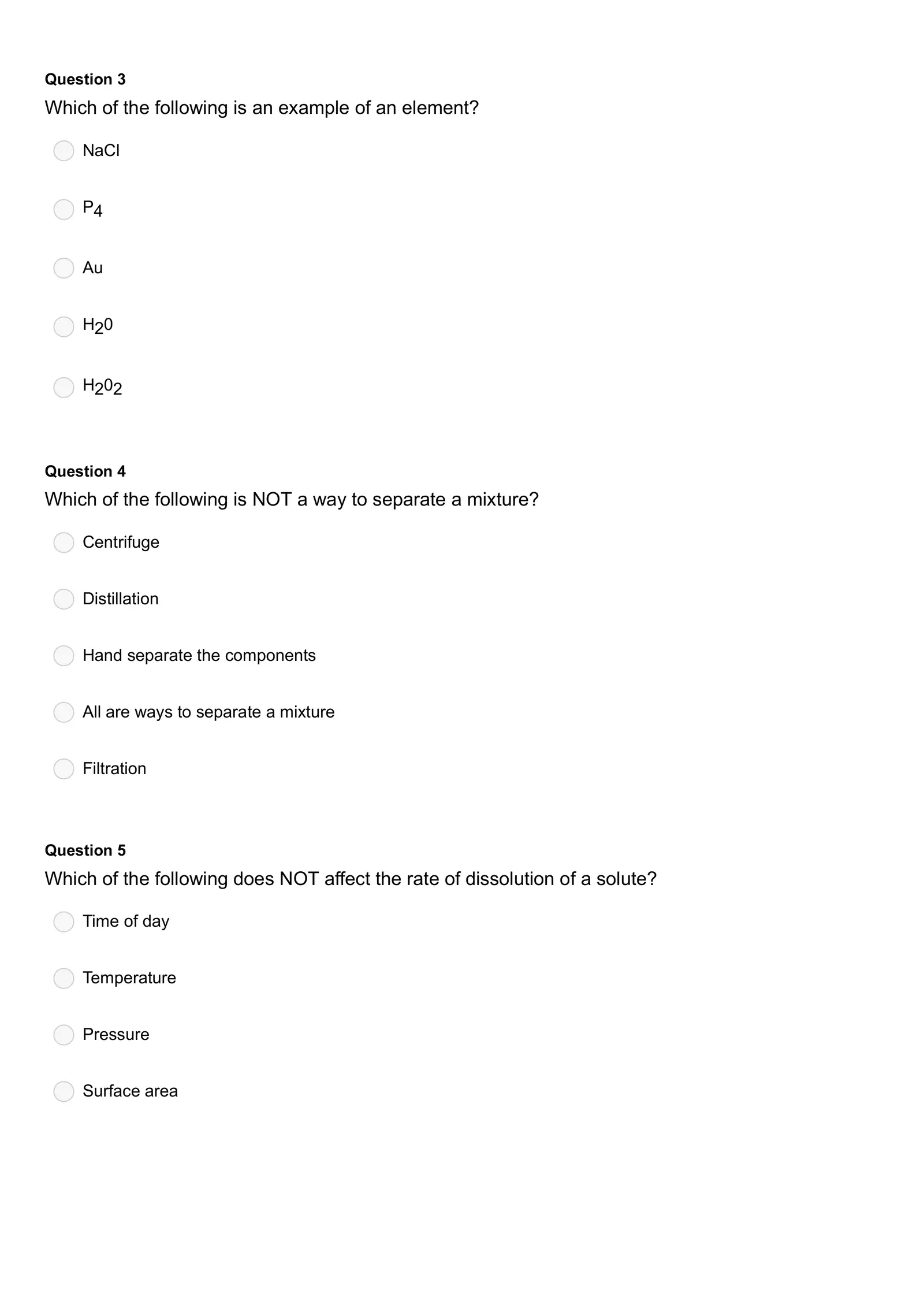Question 3 Which of the following is an example of an element? NaCl P4 Au H20 H202 Question 4 Which of the following is NOT a way to separate a mixture? Centrifuge Distillation Hand separate the components All are ways to separate a mixture Filtration Question 5 Which of the following does NOT affect the rate of dissolution of a solute? Time of day Temperature Pressure Surface area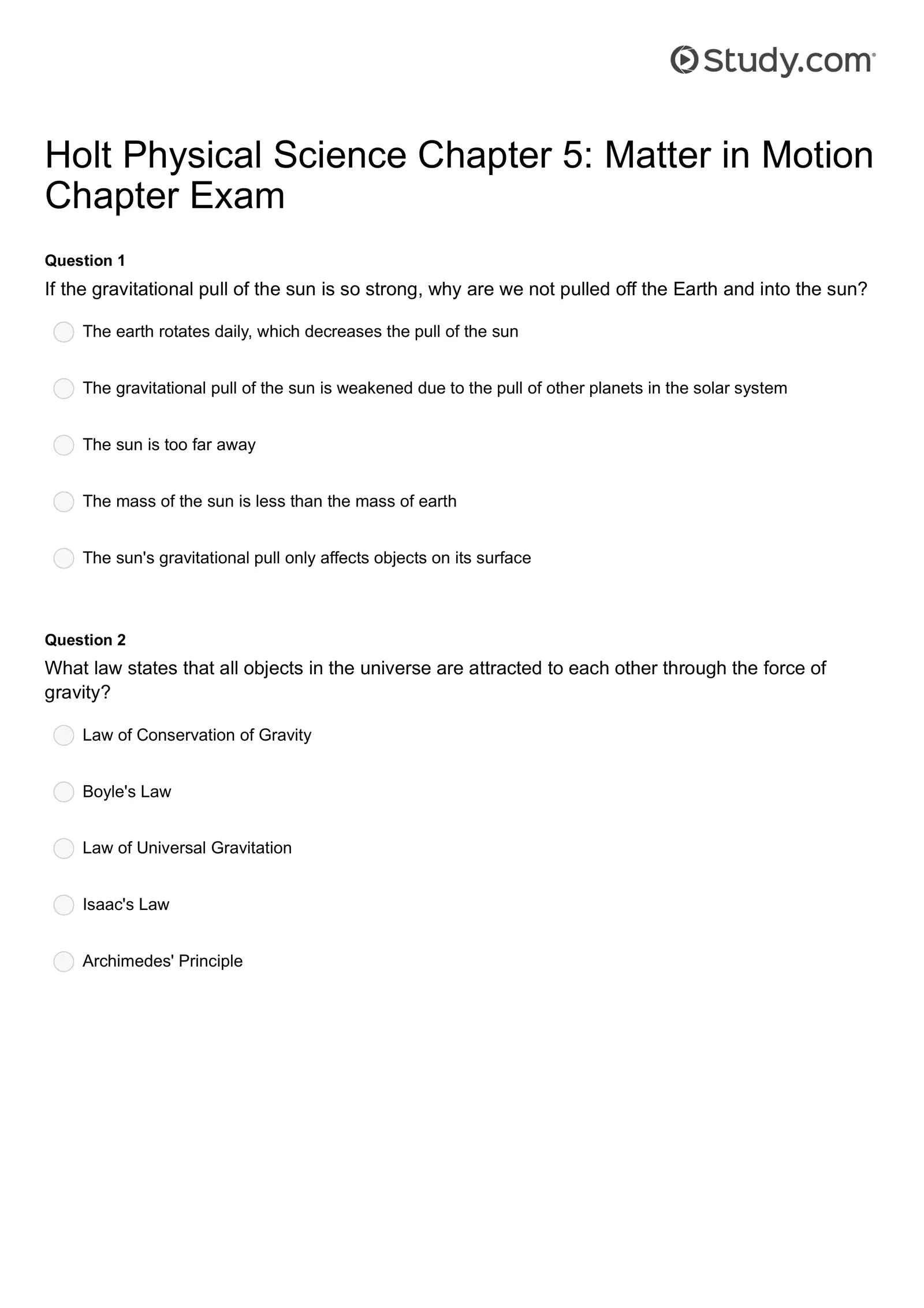Holt Physical Science Chapter 5: Matter in Motion Chapter Exam Question 1 If the gravitational pull of the sun is so strong, why are we not pulled off the Earth and into the sun? The earth rotates daily, which decreases the pull of the sun The gravitational pull of the sun is weakened due to the pull of other planets in the solar system The sun is too far away The mass of the sun is less than the mass of earth The sun's gravitational pull only affects objects on its surface Question 2 What law states that all objects in the universe are attracted to each other through the force of gravity? Law of Conservation of Gravity Boyle's Law Law of Universal Gravitation Isaac's Law Archimedes' Principle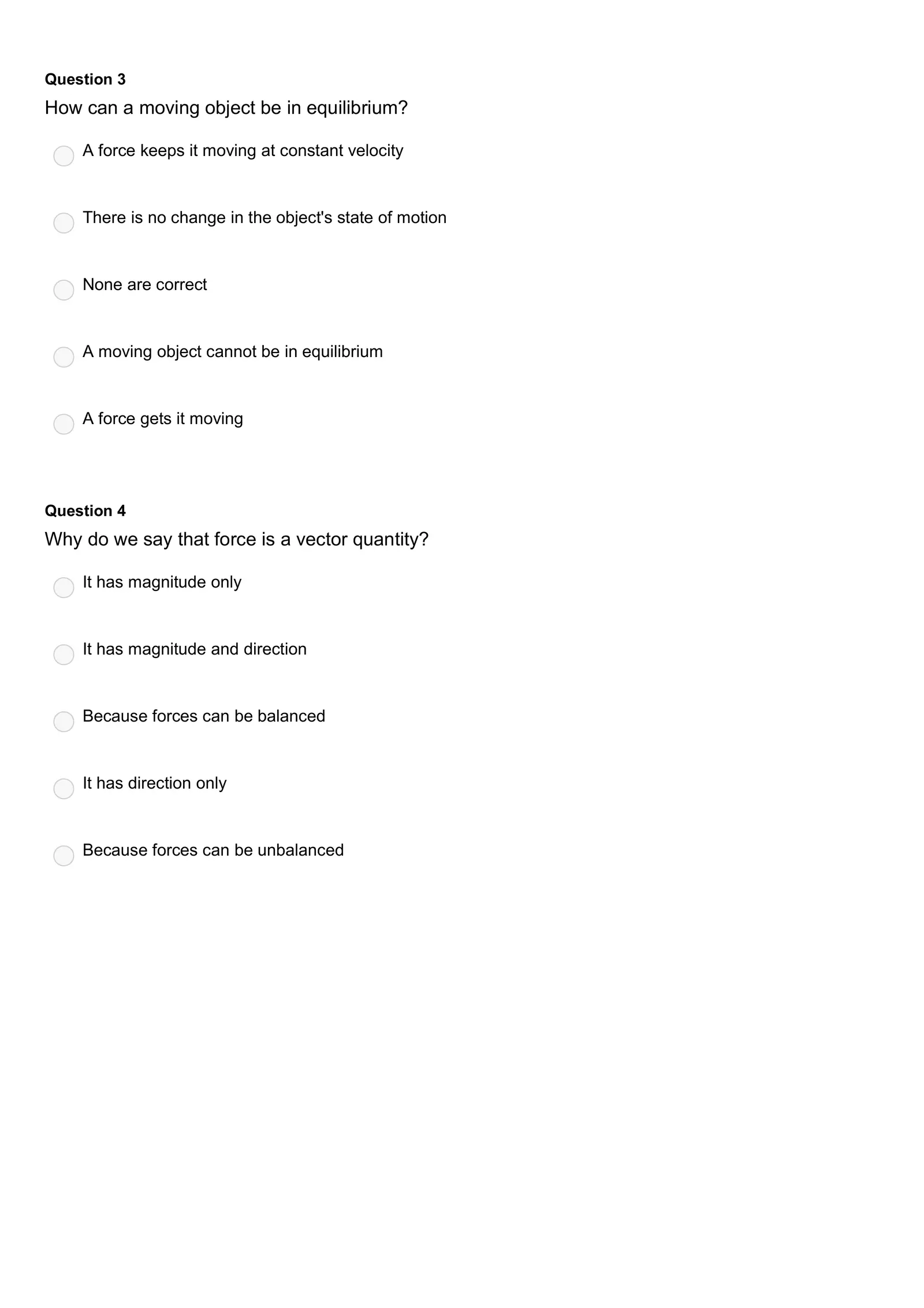Question 3 How can a moving object be in equilibrium? A force keeps it moving at constant velocity There is no change in the object's state of motion None are correct A moving object cannot be in equilibrium A force gets it moving Question 4 Why do we say that force is a vector quantity? It has magnitude only It has magnitude and direction Because forces can be balanced It has direction only Because forces can be unbalanced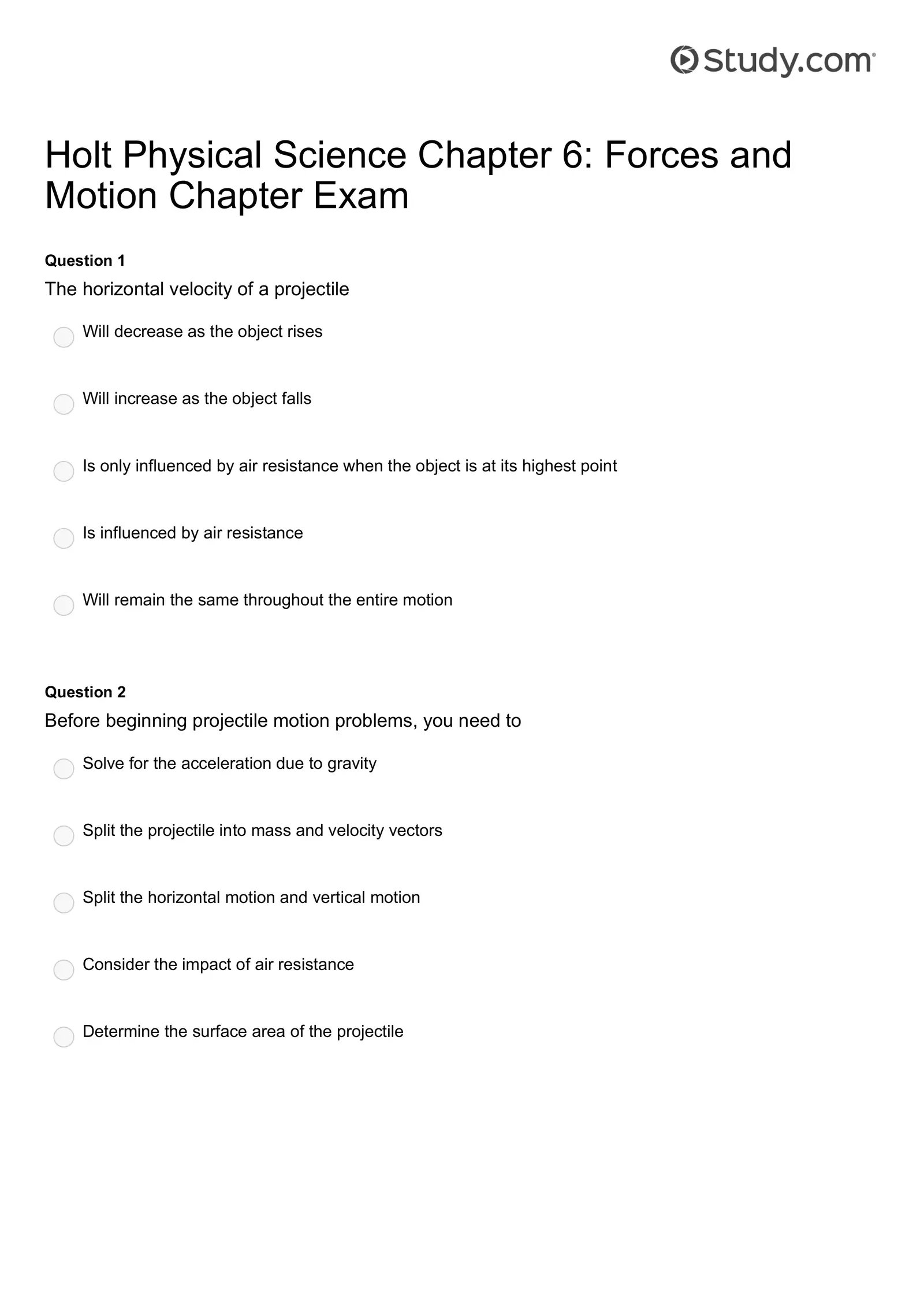Holt Physical Science Chapter 6: Forces and Motion Chapter Exam Question 1 The horizontal velocity of a projectile Will decrease as the object rises Will increase as the object falls Is only influenced by air resistance when the object is at its highest point Is influenced by air resistance Will remain the same throughout the entire motion Question 2 Before beginning projectile motion problems, you need to Solve for the acceleration due to gravity Split the projectile into mass and velocity vectors Split the horizontal motion and vertical motion Consider the impact of air resistance Determine the surface area of the projectile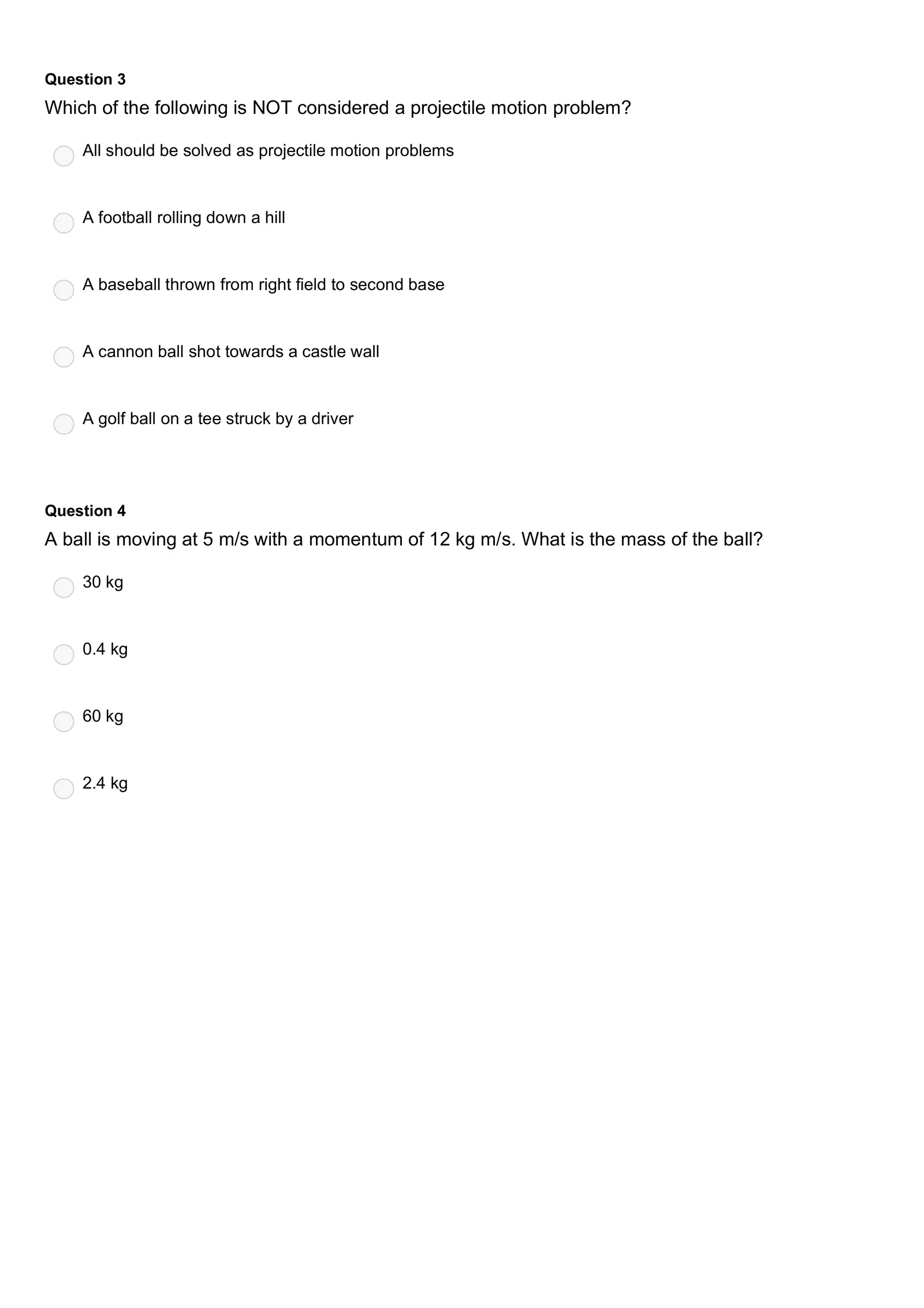Question 3 Which of the following is NOT considered a projectile motion problem? All should be solved as projectile motion problems A football rolling down a hill A baseball thrown from right field to second base A cannon ball shot towards a castle wall A golf ball on a tee struck by a driver Question 4 A ball is moving at 5 m/s with a momentum of 12 kg m/s. What is the mass of the ball? 30 kg 0.4 kg 60 kg 2.4 kg